# CREST Reasoning Olympiad Class 3 Sample Papers

REGISTER NOW

## Syllabus:

Section 1: Patterns, Analogy and Classification, Geometrical Shapes, Mirror Images and Water Images, Direction Sense Test, Ranking Test, Alphabet Test and Logical Sequence of Words, Puzzle Test, Coding - Decoding.

Achievers Section: Higher Order Thinking Questions - Syllabus as per Section 1

 Q.1 The following figure shows four boxes with different books in them. Which of the following box contains a GK book but no Hindi book?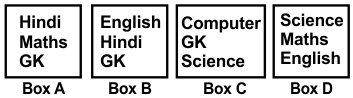Q.2 Find out the next figure of the following series: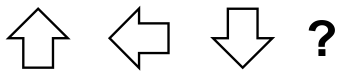Q.3 Which of the following will be the correct water image of the given figure?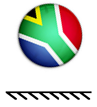Q.4 Which of the following will be the correct mirror image of the given figure?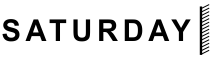Q.5 The following question consists of two words each that have a certain relationship with each other. Select the pair that has the same relationship as the original words: Spider : Web
 Q.6 How many triangles are there in the given figure?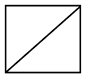Q.7 If A = 1, C = 3, then F = ?
 Q.8 If MONDAY = 1, TUESDAY = 2, then THURSDAY = ?
 Q.9 In a certain code, PIZZA is coded as #@##@, BURGER is coded as #@##@#, then TOFFEE will be coded as:
 Q.10 If in a certain code language 'SYSTEM' is written as 'SYSMET', 'NEARER' is written as 'AENRER', then how will 'FRACTION' be written in that language?

Sample PDF of CREST Reasoning Olympiad for Class 3: